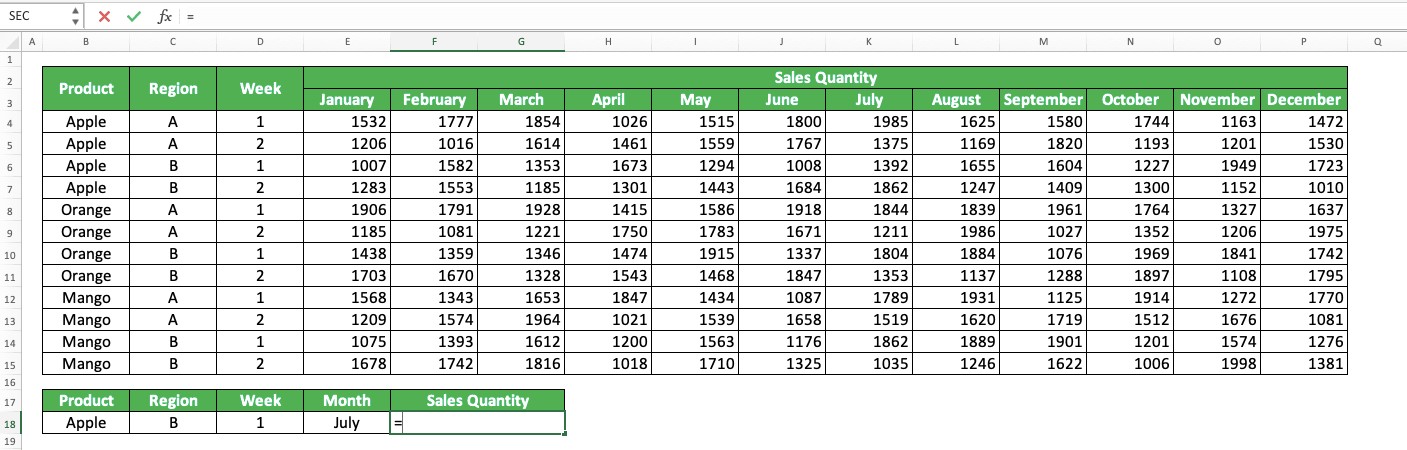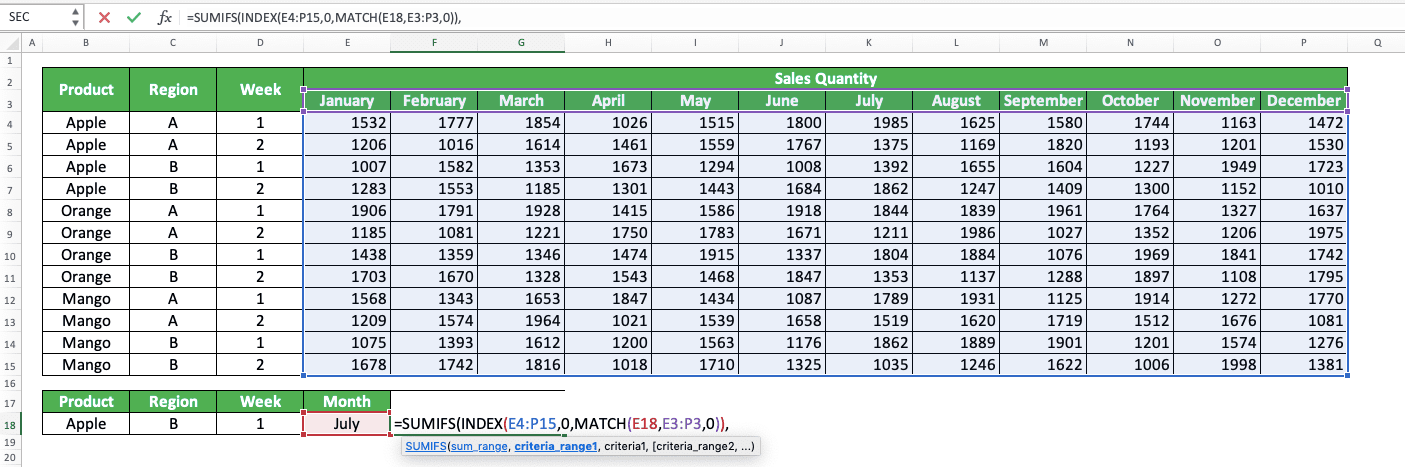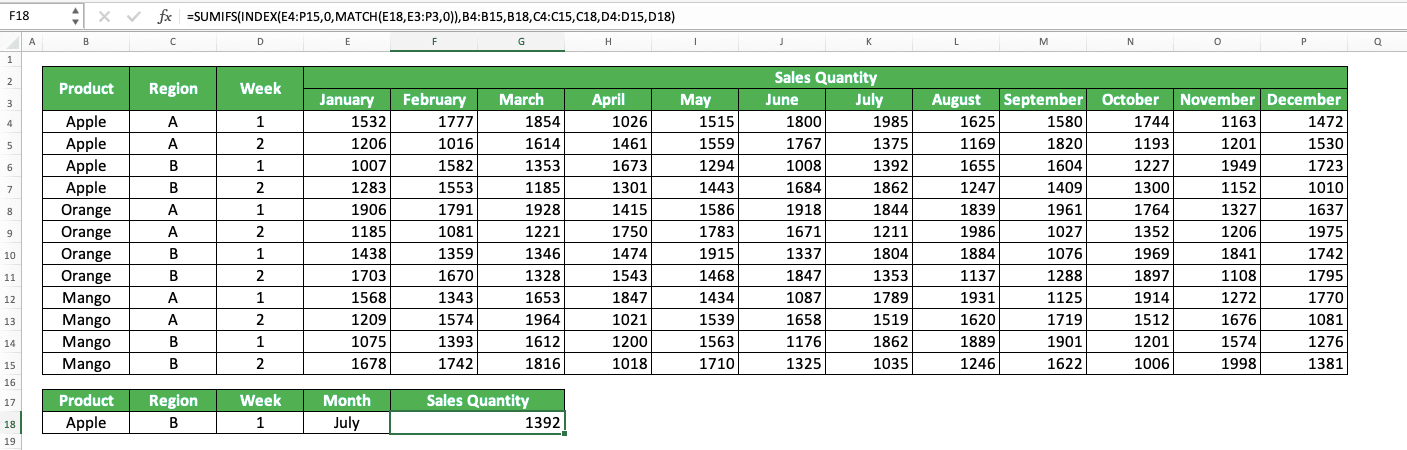How to Combine SUMIFS with INDEX MATCH - Compute Expert

# How to Combine SUMIFS with INDEX MATCH

Home >> Excel Tutorials from Compute Expert >> Excel Formulas List >> How to Combine SUMIFS with INDEX MATCH

In this tutorial, you will learn how to combine SUMIFS and INDEX MATCH in excel completely.

When working in excel, we might sometimes need to find a specific number in our data table with multiple lookup references. If we know how to use this SUMIFS and INDEX MATCH combination, then we can do that task fast.

Want to know more about this SUMIFS and INDEX MATCH combination and master the way to use it in excel? Read this tutorial until its last part!

Disclaimer: This post may contain affiliate links from which we earn commission from qualifying purchases/actions at no additional cost for you. Learn more

## Why do We Combine SUMIFS with INDEX MATCH?

There are two possible reasons why we want to combine SUMIFS with INDEX MATCH in Excel. First, we want to look up a number with multiple lookup criteria. Second, we want to sum numbers from data entries that fulfill specific criteria.

If we have just one specific column/row from where we want to get those numbers, we can just use SUMIFS.

However, if we want the column/row of those numbers to be more flexible, we should combine SUMIFS with INDEX MATCH. The INDEX MATCH will act as the determinant of that column/row according to the criterion we have.

## The Way to Write It and Its Inputs

Here is the general writing form of SUMIFS INDEX MATCH in excel to achieve our purpose when using it.

= SUMIFS ( INDEX ( all_numbers_range , 0 , MATCH ( number_column_header , all_numbers_column_header_row_range , 0 ) ) , data_range1 , criterion1 , data_range2 , criterion2 , … )

In this writing, we assume that the numbers in our data table are separated by columns. That is why we write our MATCH in our INDEX column number input part. If your numbers are separated by rows, just write your MATCH in the INDEX row number input part (switch it with the 0).

We write INDEX MATCH as the number range input of our SUMIFS because we want it to determine our number range. In the INDEX, we input the cell range where all the numbers we possibly want to look up are.

Next, we input 0 as our INDEX row number. That is because we want to get the cell range of all the rows of the column we select with MATCH.

In our MATCH, we input the number column header we want as its first input. We usually input a cell here so we can be flexible with the column we want to get our number from.

We also input the row cell range where all our number column headers are. We input 0 as the search mode because we want an exact match for our desired number column header.

After we write the INDEX MATCH, we input all the data ranges which data we want to evaluate with our criteria. We also input the corresponding criterion after each of the data range we input. We input them just as we usually do when we input sum criteria to our SUMIFS.

By doing all that, we will write the SUMIFS and INDEX MATCH combination that can find our number with multiple criteria!

## Example of Its Usage and Result

To make the SUMIFS INDEX MATCH concept clearer, here is its implementation example in excel.As you can see there, we can get our number or sum of numbers according to multiple lookup criteria. We can do that by combining SUMIFS with INDEX MATCH in the way we have discussed in the previous section.

As our sales quantities are separated by columns here, we write our MATCH in the column index input of our INDEX. We input the cell range where all our sales quantities reside first in INDEX before we input 0 and our MATCH.

In the MATCH, we input the cell that contains the month of the sales quantity we look for. Then, we input the row cell range of the month headers and 0 as the search mode of our MATCH.

After the INDEX MATCH, we input the columns where we evaluate our sales quantities criteria plus the criteria themselves. For the third sales quantity, we want to sum the week 1 sales quantities in March. Therefore, we input only the month column and the “March” criterion there.

We do all that and we immediately get the sales quantities we need by combining SUMIFS and INDEX MATCH!

## Writing Steps

After we have discussed the function, way of writing, and implementation example of SUMIFS INDEX MATCH, let’s discuss its writing steps. This formulas combination is a little complex so read the steps multiple times if you have trouble understanding them!

Note: These writing steps assume your numbers are separated by columns like a common data table. If your numbers are separated by rows, place your MATCH writing in the INDEX row number input part.

1. Type an equal sign ( = ) in the cell where you want to put your SUMIFS INDEX MATCH result2. Type SUMIFS (can be with large letters or small letters) and an open bracket sign after =3. Type INDEX (can be with large letters or small letters) and an open bracket sign4. Input the cell range where all the numbers you potentially have to find/sum are. Then, type a comma sign ( , )5. Type 0 and type a comma sign6. Type MATCH (can be with large letters or small letters) and an open bracket sign7. Input the column header of the column you want to get your number from. We usually input a cell coordinate here so the column where we get our number can be more flexible. Then, type a comma sign8. Input the row cell range where you place all the column headers of your numbers. Then, type a comma sign9. Type 0 , two close bracket signs, and a comma sign10. Input the cell range where the data you want to evaluate with your first criterion is. Then, type a comma sign11. Input the first criterion with what you want to evaluate the data in the cell range you previously inputted12. Optional: Type a comma sign and then repeat steps 10-11 until you have inputted all your criteria13. Type a close bracket sign14. Press Enter
15. Done!## Exercise

After you have learned how to combine SUMIFS with INDEX MATCH in excel, let’s do an exercise to deepen your understanding!

### Questions

Give your answer by using SUMIFS INDEX MATCH in the appropriate gray-colored cell according to the question number!
1. What is the average test score from class 2-F in region II for test 8?
2. What is the average test score from class 1-E for test 5?
3. What is the sum of the average test scores from class 3 in region II for test 10?

You can search for a number with up to 127 lookup criteria if you combine SUMIFS with INDEX MATCH.

Related tutorials you should learn from:

Get updated excel info from Compute Expert by registering your email. It's free!